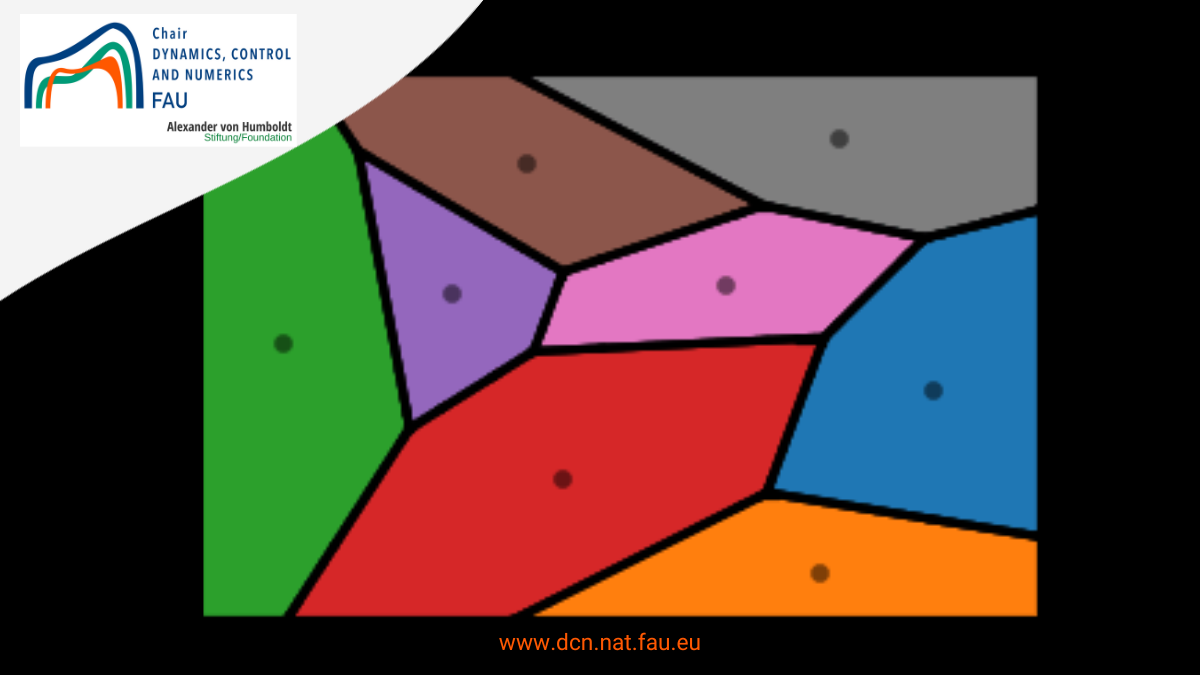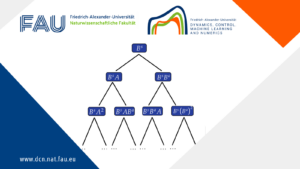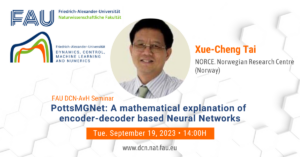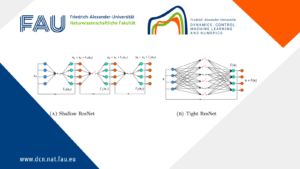# Lloyd’s AlgorithmIn this repository, we show a code for Lloyd’s algorithm.

Also called Voronoid iteration, this is an iterative algorithm finding for equispaced convex cells in euclidean space.

Lloyd’s algorithm finds the distribution of the cells computing their center of mass and iteratively applying the Voronoid tessellation. Like the closely related K-means clustering algorithm, it repeatedly finds the centroid of each set in the partition and then re-partitions the input according to which of these centroids is closest.

After performing this iterative process, we can visualize how Lloyd’s algorithm converges to obtain an equispaced distribution of convex cells.

In the code you can find different functions, using to compute the Voronoid diagram, to compute the center of mass, and to execute al the algoritm.

In the last part of the code you can find the part of “Setting”, in this place you can change the number of iteration, the number of cell that you want etc.

### You might like!#### FAU MoD Lecture: Free boundary regularity for the obstacle problem#### Stability of hyperbolic systems with non-symmetric partial dissipation#### PottsMGNet: A Mathematical Explanation of Encoder-Decoder Based Neural Networks